### IMO Shortlist 2002 problem A6

Kvaliteta:
Avg: 3,0
Težina:
Avg: 8,0
Let$A$ be a non-empty set of positive integers. Suppose that there are positive integers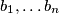$b_1,\ldots b_n$ and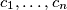$c_1,\ldots,c_n$ such that

- for each$i$ the set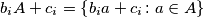$b_iA+c_i=\left\{b_ia+c_i\colon a\in A\right\}$ is a subset of$A$, and

- the sets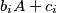$b_iA+c_i$ and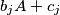$b_jA+c_j$ are disjoint whenever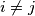$i\ne j$

Prove that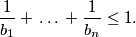Izvor: Međunarodna matematička olimpijada, shortlist 2002